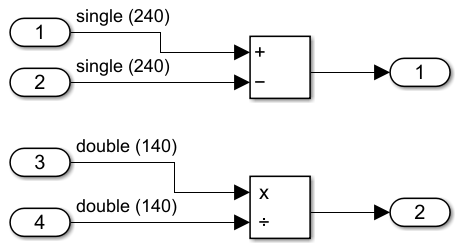Main Content

## Generate SIMD Code from Simulink Blocks

You can generate SIMD (single instruction, multiple data) code from certain Simulink blocks by using Intel AVX and Intel SSE technology. SIMD is a computing paradigm in which a single instruction processes multiple data. Many modern processors have SIMD instructions that, for example, perform several additions or multiplications at once. For computationally intensive operations on supported blocks, SIMD intrinsics can significantly improve the performance of the generated code on Intel platforms.

### Blocks That Support SIMD Code Generation

When certain conditions are met, you can generate SIMD code by using Intel SE or Intel AVX technology. This table lists blocks that support SIMD code generation. The table also details the conditions under which the support is available.

BlockConditions
Add
• For Intel AVX and Intel SSE, the input signal has a data type of `single`, `double`, `int32`, or `int64`.

• For Intel AVX-512, the input signal has a data type of `single` or `double`.

Subtract
• For Intel AVX and Intel SSE, the input signal has a data type of `single`, `double`, `int32`, or `int64`.

• For Intel AVX-512, the input signal has a data type of `single` or `double`.

Product
• For Intel AVX and Intel SSE, the input signal has a data type of `single`, `double`, or `int32`.

• For Intel AVX-512, the input signal has a data type of `single` or `double`.

• Set Multiplication parameter to `Element-wise(.*)`

Gain
• For Intel AVX or Intel SSE, the input signal has a data type of `single`, `double`, or `int32`.

• For Intel AVX-512, the input signal has a data type of `single` or `double`.

• Set Multiplication parameter to `Element-wise(.*)`

DivideThe input signal has a data type of `single` or `double`.
SqrtThe input signal has a data type of `single` or `double`.
Ceil
• For Intel SSE and Intel AVX, the input signal has a data type of `single` or `double`.

• Intel AVX-512 is not supported.

Floor
• For Intel SSE and Intel AVX, the input signal has a data type of `single` or `double`.

• Intel AVX-512 is not supported.

MinMax
• The input signal has a data type of `single` or `double`.

• The value of the Support: non-finite numbers configuration parameter is set to `off`.

MATLAB FunctionMATLAB code meets the conditions specified in this topic: Generate SIMD Code for MATLAB Functions.
For Each Subsystem
• The For Each Subsystem block contains a block listed in this table that meets the specified conditions.

• The value of the Partition Dimension block parameter must be above the value of the Loop unrolling threshold configuration parameter.

If you have DSP System Toolbox™, you can also generate SIMD code from certain DSP System Toolbox blocks. For more information, see Simulink Blocks in DSP System Toolbox that Support SIMD Code Generation (DSP System Toolbox).

### Generate SIMD Code Compared to Plain C Code

The simple model `simdDemo` has a Subtract block and a Divide block. The Subtract block has an input signal that has a dimension of 240 and an input data type of `single`. The Divide block has an input signal that has a dimension of 140 and an input data type of `double`.The plain generated C code for this model is:

```void simdDemo_step(void) { int32_T i; for (i = 0; i < 240; i++) { simdDemo_Y.Out1[i] = simdDemo_U.In1[i] - simdDemo_U.In2[i]; } for (i = 0; i < 140; i++) { simdDemo_Y.Out2[i] = simdDemo_U.In3[i] / simdDemo_U.In4[i]; } }```
In the plain (non-SIMD) C code, each loop iteration produces one result.

To generate SIMD code

1. Open the Embedded Coder app.

2. Click Settings > Hardware Implementation.

3. Set the Device vendor parameter to `Intel` or `AMD`.

4. Set the Device type parameter to `x86-64(Windows 64)` or `x86-64(Linux 64)`.

5. On the Interface pane, for the Code replacement libraries parameter, click Select. In the dialog box that opens, choose an Intel AVX or Intel SSE library. The library that you choose depends on which instruction set extension your processor supports. For more information, see https://www.intel.com/content/www/us/en/support/articles/000005779/processors.html. This table lists which Intel intrinsic instructions sets each code replacement library contains:

Code Replacement LibraryIntel Intrinsic Instruction Set
Intel SSESSE, SSE2, SSE4.1
Intel AVXSSE, SSE2, SSE4.1, AVX, AVX2
Intel AVX-512SSE, SSE2, SSE4.1, AVX, AVX2, AVX-512

6. Generate code from the model.

```void simdDemo_step(void) { int32_T i; for (i = 0; i <= 236; i += 4) { _mm_storeu_ps(&simdDemo_Y.Out1[i], _mm_sub_ps(_mm_loadu_ps(&simdDemo_U.In1[i]), _mm_loadu_ps(&simdDemo_U.In2[i]))); } for (i = 0; i <= 138; i += 2) { _mm_storeu_pd(&simdDemo_Y.Out2[i], _mm_div_pd(_mm_loadu_pd(&simdDemo_U.In3[i]), _mm_loadu_pd(&simdDemo_U.In4[i]))); } } ```

This code is for the `Intel SSE` code replacement library. The SIMD instructions are the intrinsic functions that start with the identifier `_mm`. These functions process multiple data in a single iteration of the loop because the loop increments by four for single data types and by two for double data types. For models that process more data and are computationally more intensive than this one, the presence of SIMD instructions can significantly speed up the code execution time.

For a list of a Intel intrinsic functions for supported Simulink blocks, see https://software.intel.com/sites/landingpage/IntrinsicsGuide/.

### Limitations

The generated code is not optimized through SIMD if:

• The code in a MATLAB Function block contains scalar data types outside the body of loops. For instance, if `a,b`, and `c` are scalars, the generated code does not optimize an operation such as `c=a+b`.

• The code in a MATLAB Function block contains indirectly indexed arrays or matrices. For instance if `A,B,C`, and `D` are vectors, the generated code is not vectorized for an operation such as `D(A)=C(A)+B(A)`.

• The blocks within a reusable subsystem might not be optimized.

• If the code in a MATLAB Function block contains parallel for-Loops (`parfor`), the `parfor` loop is not optimized with SIMD code, but loops within the body of the `parfor` loop can be optimized with SIMD code.

Download guide